Question

Rigid rods of negligible mass lying along the y axisconnect three particles (Fig. P10.20). The system rotates about thex axis with an angular speedof 3.00 rad/s.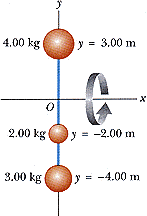Figure P10.20

(a) Find the moment of inertia about thex axis.
kg·m2
Find the total rotational kinetic energy evaluated from 1/2I2.
J
(b) Find the tangential speed of each particle.
m/s (4.00 kgparticle)
m/s (2.00 kgparticle)
m/s (3.00 kgparticle)
Find the total kinetic energy evaluated from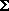mivi2/2.
J

We need at least 10 more requests to produce the answer.

0 / 10 have requested this problem solution

The more requests, the faster the answer.

All students who have requested the answer will be notified once they are available.

Earn Coins

Coins can be redeemed for fabulous gifts.

Similar Homework Help Questions
• step by step. box in answer please Rigid rods of negligible mass lying along the y...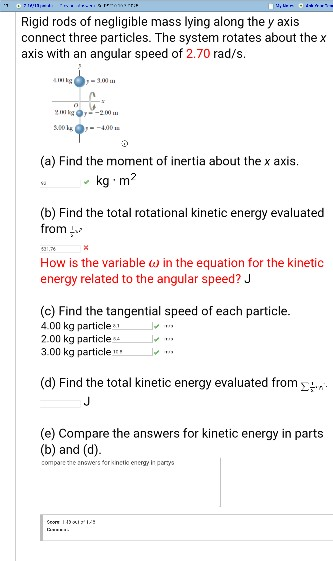step by step. box in answer please Rigid rods of negligible mass lying along the y axis connect three particles. The system rotates about the x axis with an angular speed of 2.70 rad/s (a) Find the moment of inertia about the x axis kg m (b) Find the total rotational kinetic energy evaluated from How is the variable ω in the equation for the kinetic energy related to the angular speed? J (c) Find the tangential speed of each...

• rods of negligible mass lying along the y axis connect three particles. The system rotates about...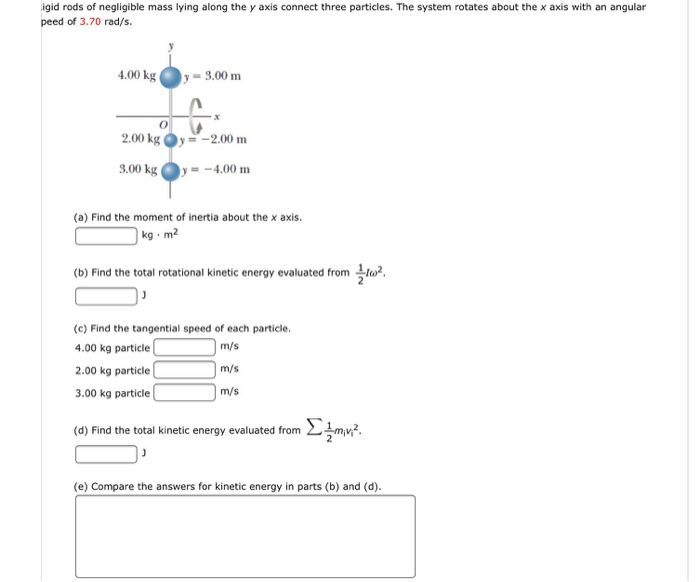rods of negligible mass lying along the y axis connect three particles. The system rotates about the x axis with an angular peed of 3.70 rad/s. (a) Find the moment of inertia about the x axis. kg middot m^2 (b) Find the total rotational kinetic energy evaluated from 1/2 I omega^2. J (c) Find the tangential speed of each particle. 4.00 kg particle m/s 2.00 kg particle m/s 3.00 kg particle m/s (d) Find the total kinetic energy evaluated from...

• Intertia and Rotational Energy Quick Question!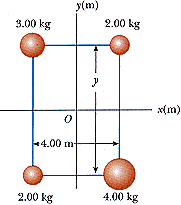The four particles shown below are connected by rigid rods ofnegligible mass where y = 5.90 m. The origin is at the center of therectangle.(a) If the system rotates in the xyplane about the z axis with an angular speed of 6.00rad/s, calculate the moment of inertia of the system aboutthez axis.kg·m2(b) Calculate the rotational energy of the system. J

• 1a. 1b. A disk with a rotational inertia of 1.20 kg·m2 rotates like a merry-go-round while...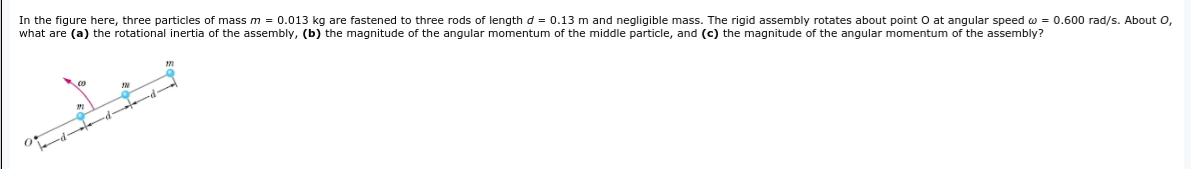1a. 1b. A disk with a rotational inertia of 1.20 kg·m2 rotates like a merry-go-round while undergoing a torque given by τ = (4.01 + 2.79t) N · m. At time t = 1.00 s, its angular momentum is 3.53 kg·m2/s. What is its angular momentum at t = 3.00 s? In the figure here, three particles of mass m = 0.013 kg are fastened to three rods of length d = 0.13 m and negligible mass. The rigid assembly...

• Rigid rods of negligible mass lying along the y axis connect three particles. The system rotates...

Rigid rods of negligible mass lying along the y axis connect three particles. The system rotates about the x axis with anangular speed of 1.50 rad/s.(b) Find the tangential speed of each particle.m/s (4.00 kg particle)m/s (2.00 kg particle)m/s (3.00 kg particle)

• The four particles shown below are connected by rigid rods of negligible mass where y1 = 6.60 m.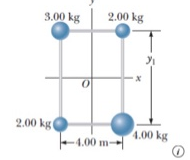The four particles shown below are connected by rigid rods of negligible mass where y1 = 6.60 m. The origin is at the center of the rectangle. The system rotates in the xy plane about the z axis with an angular speed of 6.40 rad/s. (a) Calculate the moment of inertia of the system about the z axis.(b) Calculate the rotational kinetic energy of the system.

• The four particles shown below are connected by rigid rods of negligible mass where y1 = 6.60 m.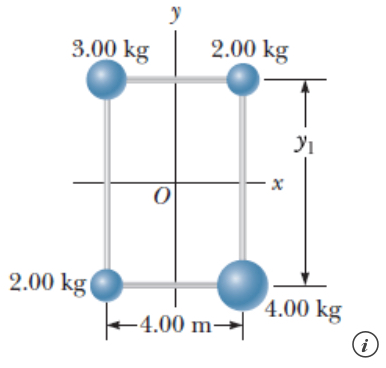The four particles shown below are connected by rigid rods of negligible mass where y1 = 6.60 m. The origin is at the center of the rectangle. The system rotates in the xy plane about the z axis with an angular speed of 5.40 rad/s. (a) Calculate the moment of inertia of the system about the z axis.(b) Calculate the rotational kinetic energy of the system.

• The four particles shown below are connected by rigid rods of negligible mass where y1 = 6.60 m

The four particles shown below are connected by rigid rods of negligible mass where y1 = 6.60 m. The origin isat the center of the rectangle. The system rotates in the xy plane about the z axis with an angular speed of 5.70rad/s.(b) Calculate the rotational kinetic energy of the system.

• 2. A bar on a hinge starts from rest and rotates with an angular acceleration α...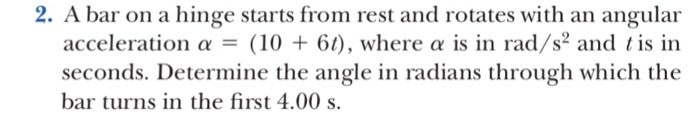2. A bar on a hinge starts from rest and rotates with an angular acceleration α (10 + 61), where α is in rad/s" and 1 is in seconds. Determine the angle in radians through which the bar turns in the first 4.00 s. 4. A dentist's drll starts from rest. After 3.20 s of constant an- gular acceleration, it turns at a rate of 2.51 × 104 rev/min. (a) Find the drill's angular acceleration. (b) Determine the angle (in...